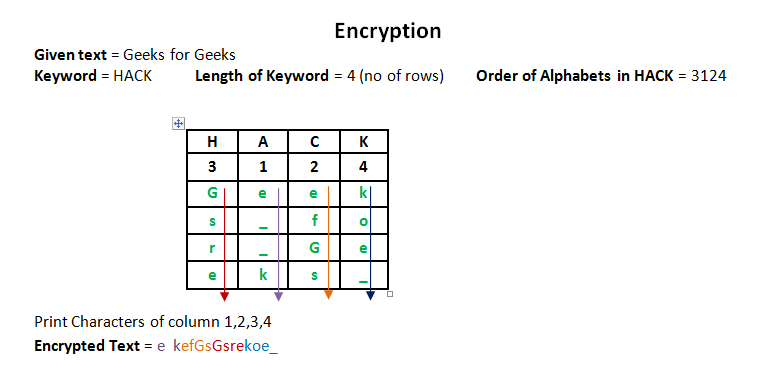Related Articles
Columnar Transposition Cipher
• Difficulty Level : Medium
• Last Updated : 30 Aug, 2019

Given a plain-text message and a numeric key, cipher/de-cipher the given text using Columnar Transposition Cipher

The Columnar Transposition Cipher is a form of transposition cipher just like Rail Fence Cipher. Columnar Transposition involves writing the plaintext out in rows, and then reading the ciphertext off in columns one by one.

Examples:

```Encryption
Input : Geeks for Geeks
Key = HACK
Output : e  kefGsGsrekoe_
Decryption
Input : e  kefGsGsrekoe_
Key = HACK
Output : Geeks for Geeks

Encryption
Input :  Geeks on work
Key = HACK
Output : e w_eoo_Gs kknr_
Decryption
Input : e w_eoo_Gs kknr_
Key = HACK
Output : Geeks on work
```

## Recommended: Please try your approach on {IDE} first, before moving on to the solution.

Encryption

In a transposition cipher, the order of the alphabets is re-arranged to obtain the cipher-text.

1. The message is written out in rows of a fixed length, and then read out again column by column, and the columns are chosen in some scrambled order.
2. Width of the rows and the permutation of the columns are usually defined by a keyword.
3. For example, the word HACK is of length 4 (so the rows are of length 4), and the permutation is defined by the alphabetical order of the letters in the keyword. In this case, the order would be “3 1 2 4”.
4. Any spare spaces are filled with nulls or left blank or placed by a character (Example: _).
5. Finally, the message is read off in columns, in the order specified by the keyword.Decryption

1. To decipher it, the recipient has to work out the column lengths by dividing the message length by the key length.
2. Then, write the message out in columns again, then re-order the columns by reforming the key word.

## C++

 `// CPP program for illustrating``// Columnar Transposition Cipher``#include``using` `namespace` `std;`` ` `// Key for Columnar Transposition``string ``const` `key = ``"HACK"``; ``map<``int``,``int``> keyMap;`` ` `void` `setPermutationOrder()``{             ``    ``// Add the permutation order into map ``    ``for``(``int` `i=0; i < key.length(); i++)``    ``{``        ``keyMap[key[i]] = i;``    ``}``}`` ` `// Encryption ``string encryptMessage(string msg)``{``    ``int` `row,col,j;``    ``string cipher = ``""``;``     ` `    ``/* calculate column of the matrix*/``    ``col = key.length(); ``     ` `    ``/* calculate Maximum row of the matrix*/``    ``row = msg.length()/col; ``     ` `    ``if` `(msg.length() % col)``        ``row += 1;`` ` `    ``char` `matrix[row][col];`` ` `    ``for` `(``int` `i=0,k=0; i < row; i++)``    ``{``        ``for` `(``int` `j=0; j::iterator ii = keyMap.begin(); ii!=keyMap.end(); ++ii)``    ``{``        ``j=ii->second;``         ` `        ``// getting cipher text from matrix column wise using permuted key``        ``for` `(``int` `i=0; i::iterator ii=keyMap.begin(); ii!=keyMap.end(); ++ii)``        ``ii->second = index++;`` ` `    ``/* Arrange the matrix column wise according ``    ``to permutation order by adding into new matrix */``    ``char` `decCipher[row][col];``    ``map<``int``,``int``>::iterator ii=keyMap.begin();``    ``int` `k = 0;``    ``for` `(``int` `l=0,j; key[l]!=``'\0'``; k++)``    ``{``        ``j = keyMap[key[l++]];``        ``for` `(``int` `i=0; i

## Python3

 `# Python3 implementation of ``# Columnar Transposition``import` `math`` ` `key ``=` `"HACK"`` ` `# Encryption``def` `encryptMessage(msg):``    ``cipher ``=` `""`` ` `    ``# track key indices``    ``k_indx ``=` `0`` ` `    ``msg_len ``=` `float``(``len``(msg))``    ``msg_lst ``=` `list``(msg)``    ``key_lst ``=` `sorted``(``list``(key))`` ` `    ``# calculate column of the matrix``    ``col ``=` `len``(key)``     ` `    ``# calculate maximum row of the matrix``    ``row ``=` `int``(math.ceil(msg_len ``/` `col))`` ` `    ``# add the padding character '_' in empty``    ``# the empty cell of the matix ``    ``fill_null ``=` `int``((row ``*` `col) ``-` `msg_len)``    ``msg_lst.extend(``'_'` `*` `fill_null)`` ` `    ``# create Matrix and insert message and ``    ``# padding characters row-wise ``    ``matrix ``=` `[msg_lst[i: i ``+` `col] ``              ``for` `i ``in` `range``(``0``, ``len``(msg_lst), col)]`` ` `    ``# read matrix column-wise using key``    ``for` `_ ``in` `range``(col):``        ``curr_idx ``=` `key.index(key_lst[k_indx])``        ``cipher ``+``=` `''.join([row[curr_idx] ``                          ``for` `row ``in` `matrix])``        ``k_indx ``+``=` `1`` ` `    ``return` `cipher`` ` `# Decryption``def` `decryptMessage(cipher):``    ``msg ``=` `""`` ` `    ``# track key indices``    ``k_indx ``=` `0`` ` `    ``# track msg indices``    ``msg_indx ``=` `0``    ``msg_len ``=` `float``(``len``(cipher))``    ``msg_lst ``=` `list``(cipher)`` ` `    ``# calculate column of the matrix``    ``col ``=` `len``(key)``     ` `    ``# calculate maximum row of the matrix``    ``row ``=` `int``(math.ceil(msg_len ``/` `col))`` ` `    ``# convert key into list and sort ``    ``# alphabetically so we can access ``    ``# each character by its alphabetical position.``    ``key_lst ``=` `sorted``(``list``(key))`` ` `    ``# create an empty matrix to ``    ``# store deciphered message``    ``dec_cipher ``=` `[]``    ``for` `_ ``in` `range``(row):``        ``dec_cipher ``+``=` `[[``None``] ``*` `col]`` ` `    ``# Arrange the matrix column wise according ``    ``# to permutation order by adding into new matrix``    ``for` `_ ``in` `range``(col):``        ``curr_idx ``=` `key.index(key_lst[k_indx])`` ` `        ``for` `j ``in` `range``(row):``            ``dec_cipher[j][curr_idx] ``=` `msg_lst[msg_indx]``            ``msg_indx ``+``=` `1``        ``k_indx ``+``=` `1`` ` `    ``# convert decrypted msg matrix into a string``    ``try``:``        ``msg ``=` `''.join(``sum``(dec_cipher, []))``    ``except` `TypeError:``        ``raise` `TypeError(``"This program cannot"``,``                        ``"handle repeating words."``)`` ` `    ``null_count ``=` `msg.count(``'_'``)`` ` `    ``if` `null_count > ``0``:``        ``return` `msg[: ``-``null_count]`` ` `    ``return` `msg`` ` `# Driver Code``msg ``=` `"Geeks for Geeks"`` ` `cipher ``=` `encryptMessage(msg)``print``(``"Encrypted Message: {}"``.``               ``format``(cipher))`` ` `print``(``"Decryped Message: {}"``.``       ``format``(decryptMessage(cipher)))`` ` `# This code is contributed by Aditya K`

Output:
```Encrypted Message: e  kefGsGsrekoe_
Decrypted Message: Geeks for Geeks
```

Try it yourself: A double columnar transposition( It was used by the U.S. Army in World War I, and it is just a columnar transposition followed by another columnar transposition).

This article is contributed by Yasin Zafar. If you like GeeksforGeeks and would like to contribute, you can also write an article using contribute.geeksforgeeks.org or mail your article to contribute@geeksforgeeks.org. See your article appearing on the GeeksforGeeks main page and help other Geeks.

Please write comments if you find anything incorrect, or you want to share more information about the topic discussed above.

Attention reader! Don’t stop learning now. Get hold of all the important DSA concepts with the DSA Self Paced Course at a student-friendly price and become industry ready.  To complete your preparation from learning a language to DS Algo and many more,  please refer Complete Interview Preparation Course.

My Personal Notes arrow_drop_up